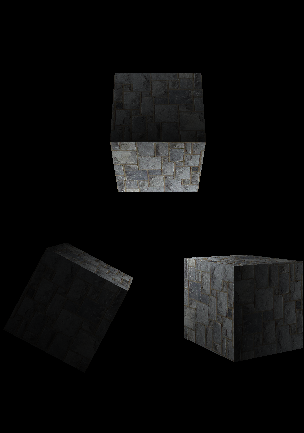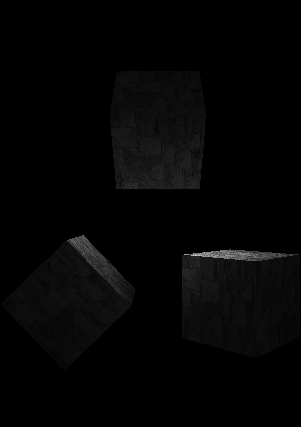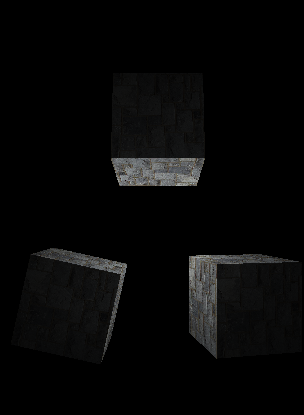# [OpenGL ES 2.0] Point Lighting gone haywire

Hello,

I’m trying to implement the fixed function pipeline from OpenGL in OpenGL ES 2. At the present moment i’m working on the point light per vertex.
However, it appears that something is not quite right. I took the shader code from the Orange Book. As you can see in the images below, the light appears to be coming from more than one place, when in fact it should be in the center between the 3 cubes.The top cube is at (0.0,2.0,0.0).
The bottom left cube is at (-2.0,-2.0,0.0).
The bottom right cube is at (2.0,-2.0,0.0).
The point light is at (0.0,0.0,0.0).
The Camera is at (0.0,0.0,10.0).

``````
precision highp float;
attribute vec4 zecubevertex;
attribute vec3 zecubenormal;
attribute vec4 zecubecolor;

varying vec4 colorVarying;
attribute vec2 zetexcoord;
varying vec2 texcoord;

struct ZECamera {
mat4 projection;
mat4 view;
mat4 mv; //ModelView
mat4 mvp;
mat4 mv_inv; //ModelView Inverse
mat4 projection_inv;
mat3 normal;
mat4 texture;
};

struct ZEMaterial{
vec4 ambient;
vec4 diffuse;
vec4 specular;
vec4 emissive;
float shininess;
};
uniform ZECamera ZEMatrixData;

uniform ZEMaterial TMaterial;

const vec4 lightPos = vec4(0.0,0.0,0.0,1.0);

void PointLight(const in vec3 normal,
const in vec3 cam_pos,
const in vec3 vertex,
inout vec4 color){
float nDotVP; //normal . light direction
float nDotHV; // normal . light halfvector
float pf; // power factor
float attenuation;
float d; //distance from surface to light source
vec3 VP; //direction from surface to light position
vec3 halfVector; // direction of maximum highlights

// Compute vector from surface to light position
VP = (ZEMatrixData.view*lightPos).xyz - vertex;
// Compute distance between surface and light position
d = length(VP);
// Normalize the vector from surface to light position
VP = normalize(VP);
// Compute attenuation
attenuation = 1.0 / (d*1.0); // linear attenuation of 0.5

halfVector = normalize(VP + cam_pos);

nDotVP = max(0.0, dot(normal, VP));
nDotHV = max(0.0, dot(normal, halfVector));
if (nDotVP == 0.0)
pf = 0.0;
else
pf = pow(nDotHV, TMaterial.shininess);

color =TMaterial.ambient * attenuation;
color += TMaterial.diffuse * nDotVP * attenuation;
color += TMaterial.specular * pf * attenuation;

}

void main()
{
gl_Position = ZEMatrixData.mvp * zecubevertex;
texcoord = zetexcoord;
vec4 ecPos = ZEMatrixData.mv * zecubevertex;
vec3 normal = normalize(zecubenormal*ZEMatrixData.normal);
PointLight(normal,-normalize(ecPos.xyz),ecPos.xyz,colorVarying );
}

``````

``````
precision highp float;
varying highp vec4 colorVarying;
varying highp vec2 texcoord;
uniform sampler2D zetexcoord;

void main()
{
highp vec4 txcl = vec4(texture2D(zetexcoord,texcoord));
gl_FragColor =  txcl * colorVarying;
}
``````

I’m clueless as to what i’m doing wrong. I’ve checked all the math calculations for the matrices, and believe these are correct. Perhaps i’m miscalculating something in the shaders?